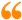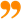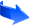﻿ Deriving the Principles of Special and general Relativity based on the Duality of Time Theory## Deriving the Principles of Special and general Relativity based on the Duality of Time Theory

This has been explained with more details in Chapter V of the Duality of Time book, as well as Chapter II of the Ultimate Symmetry. Here is a short version:

The famous Michelson-Morley experiment in 1887 proved that light travels with the same speed regardless whether it was moving in the direction of the movement of the Earth or perpendicular to it, which negated the expected aether drift effect. This led scientists to look for alternative theories, away from the luminiferous aether, to explain the nature of light and other electromagnetic phenomena. This unexpected null result initiated active research that eventually led to Special Relativity in 1905, to be generalized after ten years for non-inertial frames. The speed of light in vacuum is then considered the maximum speed which anything in the Universe can attain, regardless of the motion of the source or the inertial reference frame of the observer.

It must be noted, however, that the constancy and invariance of the speed of light is considered an axiom that has been verified experimentally but not yet proven in any theoretical sense.

Logically, there are two cases under which a quantity does not increase or decrease when we add or subtract something from it. Either this quantity in infinite, or it exists in orthogonal dimension. As we have already introduced in this article, according to the Duality of Time postulate, both these cases are equivalent and correct for the absolute speed of light in vacuum, because it is the speed of creation which is the only real speed in nature, and it is intrinsically infinite (or indefinite), but the reason why we measure a finite value of it is because of the sequential re-creation process; so individual observers are subject to the time lag during which the dimensions of space are re-created.

Moreover, since the normal time is now genuinely imaginary, the velocities of physical objects are always orthogonal onto this real and infinite speed of creation. As demonstrated in Figures III.1 and III.2 in the Ultimate Symmetry, one of the striking conclusions of the sequential re-creation in the inner levels of time is the fact that it conceives of only two primordial states: vacuum and void, whose spatial and temporal superposition is producing the multiplicity of intermediary states that appear in the cosmos as matter particles.

Vacuum is the continuous existence in the inner levels of time, and void is the discrete existence in the outer level. These two super states, which correspond to (0, 0) and (c, c) respectively, can be conceived as abstract extreme limits, but only a relative superposition of them can ever be observed or measured as relative events in space-time, or inner-outer time that is the complex-time.

As the real time flows uniformly in the inner levels, it creates the homogeneous dimensions of vacuum, and whenever it is interrupted or disturbed, it makes a new dimension that appears as a discrete instance on the outer imaginary level which is then described as void, since it does not last for more than one instance, before it is re-created again in a new state that may resemble the previous perished states, which causes the illusion of motion, while in reality it is only a result of successive discrete changes.

So the individual geometrical points can either be at rest (in the outer/imaginary time) or at the speed of creation (in the inner/real time), while the apparent limited velocities of physical particles and objects (in the total complex time, which forms the physical space-time dimensions) are the temporal average of this spatial combination that may also dynamically change as they are progressing over the outward ordinary time direction. Therefore, the Universe is always coming to be, perpetually, in "zero time" (on the outward level), and its geometrical points are sequentially fluctuating between existence and nonexistence (or vacuum and void), which means that the actual instantaneous speed of each point in space can only change from v_imaginary=0 to v_real=c, and vice versa.

This instantaneous abrupt change of speed does not contradict the laws of physics, because it is occurring in the inner levels of time before the physical objects are formed. Because they are massless, this fluctuation is the usual process that is encountered by the photons of light on the normal outward level of time, for example when they are absorbed or emitted. Hence, this model of perpetual re-creation is extending this process onto all other massive particles and objects, but on the inner levels of time where each geometrical point is still massless because it is metaphysical, while "space" and "mass" and other physical properties are actually generated from the temporal coupling, or entanglement, of these geometrical points, which is exhibited only on the outward level of time.

Accordingly, the normal limited velocity, of physical particles or objects, is a result of the spatial and temporal superposition of these dual-state velocities of its individual points N, thus: |v|=sum_N{<0|c>}/{N}, which means that 0< |v| < c.

Individually, each point is massless and it is either at rest or moving at the speed of creation, but collectively they have some non-zero inertial mass m, energy E, and limited total apparent velocity v given by the above equation.

Consequently, there is no gradual motion in the common sense that the object leaves its place to occupy new adjacent places, but it is successively re-created in those new places, i.e. motion occurs as a result of discrete change rather than infinitesimal transmutation, so the observed objects are always at rest in the different positions that they appear in.

This is the same conclusion of the Moving Arrow argument in Zeno's paradox, which Bertrand Russell described as: "It is never moving, but in some miraculous way the change of position has to occur between the instants, that is to say, not at any time whatever."

This momentous conclusion means that all frames are effectively at rest in the normal (imaginary) level of time, and there is no difference between inertial and non-inertial frames, thus there is even no need to introduce the second principle of Special Relativity (which says that the laws of physics are invariant between inertial frames) neither the equivalence principle that lead to General Relativity. These two principles, which are necessary to derive Lorentz transformations and Einstein's field equations, are implicit in the Duality of Time postulate and will follow directly from the resulting complex-time geometry as it has been shown in Chapter V of the Duality of Time book.

Furthermore, this discrete space-time structure that results from the genuinely-complex nature of time is the only way that allows exact mathematical derivation of the mass-energy equivalence relation (E=mc^2). In this manner, the Duality of Time postulate, and the resulting perpetual re-creation in the inner levels of time, can explain at once all the three principles of Special and General Relativity, and transform it into a quantum field theory because it is now based on discrete instances of dynamic space, which is the super-fluid state (c, 0) that is the ground state of matter, while the super-gas state (0, c) is the ground state of anti-matter, which accounts for super-symmetry and matter-antimatter asymmetry as we shall discuss further in this article.

### List of Some Major Problems Solved by the Duality of Time Theory

In the following articles, we will list some of the major unsolved problems in theoretical physics and describe them in brief, stating their potential solutions according to the Duality of Time Postulate. Although many of these problems will be simply eliminated according to the new genuinely-complex time-time geometry, a detailed theoretical and mathematical analysis is required in order to explain how these problems are settled. Therefore, some of the following brief suggested solutions may be speculative.

These articles are extracted from Chapter III of the Duality of Time book, and some of the details are discussed further in other chapters, as well as Volume III that is the Ultimate Symmetry. A more concise description is also published in Time Chest.

### The Duality of Time PostulateTo understand how all these major problems could be solved so easily, please study first the Duality of Time Postulate, that has been extracted from Chapter V of the Duality of Time book.

### Message from the Author:I have no doubt that this is the most significant discovery in the history of mathematics, physics and philosophy, ever!

This unique understanding of geometry will cause a paradigm shift in our knowledge of the fundamental nature of the cosmos and its corporeal and incorporeal structures.

Enjoy reading... , all the Best !

Mohamed bin Ali Haj YousefThe science of Time is a noble science, that reveals the secret of Eternity. Only the Elites of Sages may ever come to know this secret. It is called the First Age, or the Age of ages, from which time is emerging.Ibn al-Arabi [The Meccan Revelations: Volume I, page 156. - Trns. Mohamed Haj Yousef]

### Ibn al-Arabi Website:### The Meccan Revelations:### The Sun from the West:عربي Arabic

## Social SharingLike Our Facebook Page:Like Our Facebook Page:Moonlight floods the whole sky from horizon to horizon. How much it can fill your room, depends on the windows !